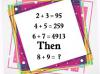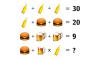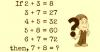BRAIN TEASERSBrain Teasers User Profile

# Brendan Chau

rank
304
points
10
See full ranking list
short ranking list
 302 Cameron Smith 10 303 Yousif Ali 10 304 Brendan Chau 10 305 Domenico Nappi 10 306 IHSAN UL HAQ INSHA MUHAMMED LATIF 10
 Can you solve this Math Puzzle? Can you solve this Math Puzzle?MATH PUZZLE: Can you replace... MATH PUZZLE: Can you replace the question mark with a number?Solve Math Puzzle If 2 + 3 = 8, 3 + 7 = 27, 4 + 5 = 32, 5 + 8 = 60, 6 + 7 = 72, then, 7 + 8 = ?